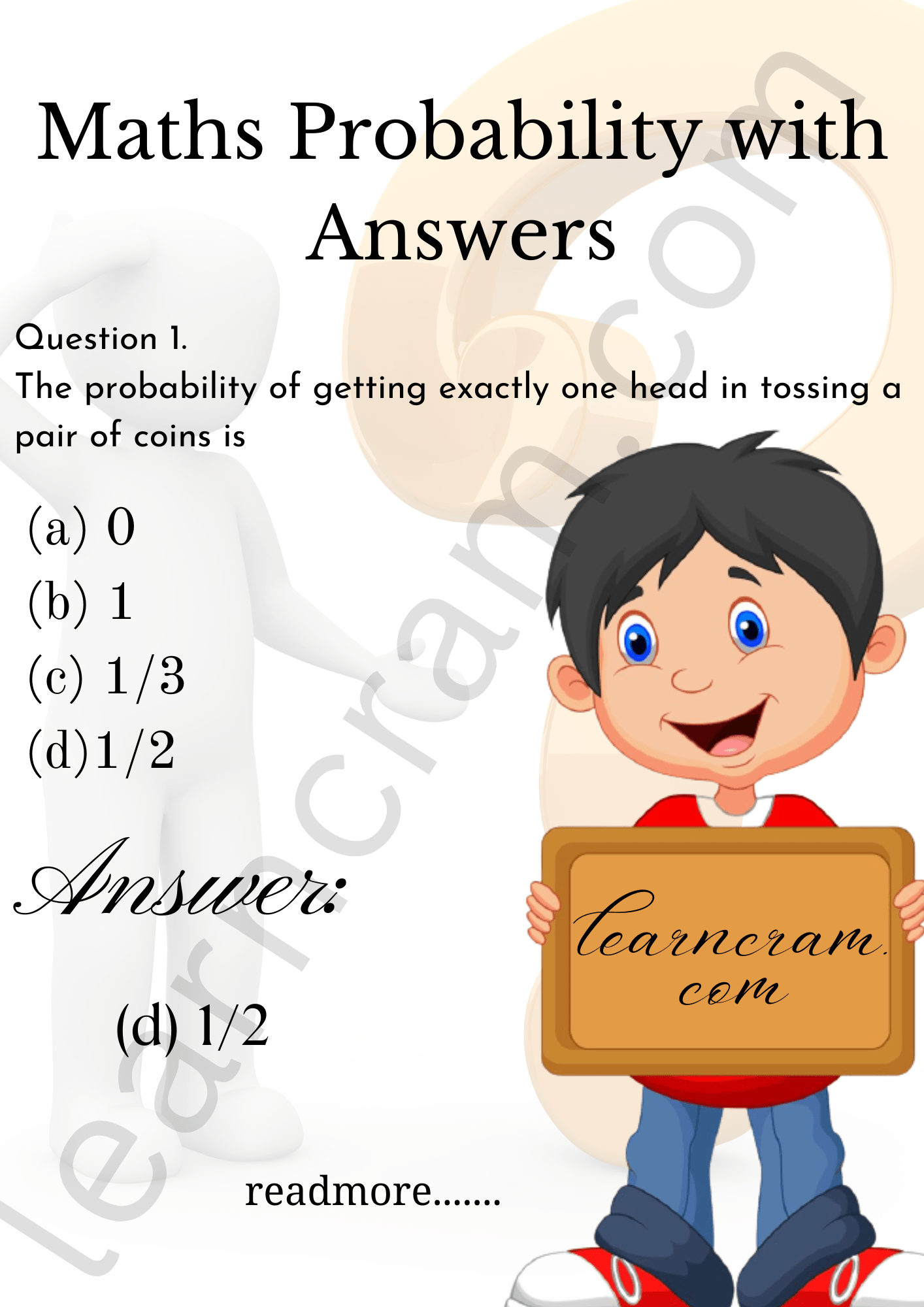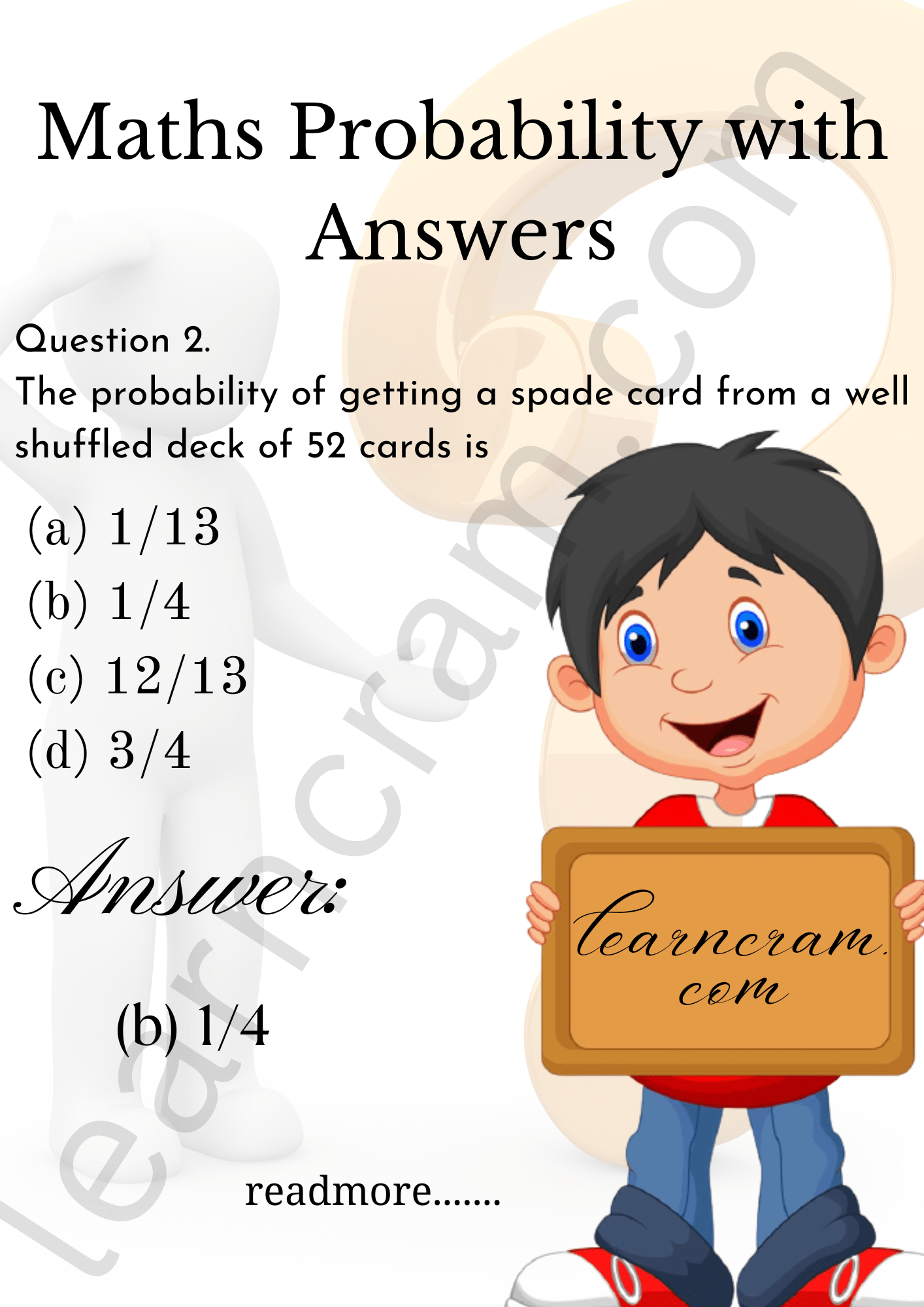# MCQ Questions for Class 10 Maths Probability with Answers

Free PDF Download of CBSE Class 10 Maths Chapter 15 Probability Multiple Choice Questions with Answers. MCQ Questions for Class 10 Maths with Answers was Prepared Based on Latest Exam Pattern. Students can solve NCERT Class 10 Maths Probability MCQs with Answers to know their preparation level.

## Class 10 Maths MCQs Chapter 15 Probability

MCQ On Probability Class 10 Question 1. The probability of getting exactly one head in tossing a pair of coins is
(a) 0
(b) 1
(c) 1/3
(d)1/2

Explaination: Reason: S = [HH, HT, TH, TT] = 4
∴ P(exactly 1 head) $$=\frac{2}{4}=\frac{1}{2}$$MCQ Questions For Class 10 Maths Probability Question 2. The probability of getting a spade card from a well shuffled deck of 52 cards isExplaination: Reason: Total cards = 52,
∴ P(a spade card) $$=\frac{13}{52}=\frac{1}{4}$$Probability MCQ Class 10 Question 3. The probability of getting less than 3 in a single throw of a die isExplaination: Reason: Here S = [1, 2, 3, 4, 5, 6]
∴ n(S) = 6
E = (Less than 3) = [1, 2
∴ P(Less than 3) $$=\frac{2}{6}=\frac{1}{3}$$

Probability Class 10 MCQ Question 4. The total number of events of throwing 10 coins simultaneously is
(a) 1024
(b) 512
(c) 100
(d) 10

Explaination: Reason: Total events 210 = 1024

MCQ Of Probability For Class 10 Question 5. Which of the following can be the probability of an event?
(a) – 0.4
(b) 1.004
(c) $$\frac{18}{23}$$
(d) $$\frac{10}{7}$$

Explaination: Reason: The probability of an event can neither be a negative value, nor it can exceed unity.

Probability MCQs With Answers Pdf Question 6. Three coins are tossed simultaneously. The probability of getting all heads isExplaination: Reason: Here S = [HHH, HHT, HTH, THH, HTT, THT, TTH, TTT] = 8
∴ P(all heads) = $$\frac{1}{8}$$

Probability MCQ Question 7. One card is drawn from a well shuffled deck of 52 cards. The probability of getting a king of red colour isExplaination: Reason: Total cards = 52
Total events «(S) = 52
a king of red colour = 2
P(a king of red colour) $$=\frac{2}{52}=\frac{1}{26}$$

MCQ Questions For Class 10 Maths With Answers Question 8. One card is drawn from a well shuffled deck of 52 playing cards. The probability of getting a non-face card isExplaination: Reason; Total cards = 52,
Total face cards = 12
∴ Non-face cards = 52 – 12 = 40
∴ P(a non-face card) $$=\frac{40}{52}=\frac{10}{13}$$

9. The chance of throwing 5 with an ordinary die isExplaination: Reason: Here S = [1, 2, 3,4, 5, 6]
∴ n(S) = 6
∴ P(throwings) = $$\frac{1}{6}$$

MCQ Questions For Class 10 Maths Question 10. The letters of the word SOCIETY are placed at random in a row. The probability of getting a vowel isExplaination: Reason: Totle letters = 7
No. of vowel = 3 [∵ Vowel are O, I, E]
∴ P(a vowel) = $$\frac{3}{7}$$

Probability Questions And Answers Pdf Question 11. Cards bearing numbers 3 to 20 are placed in a bag and mixed thoroughly. A card is taken out from the bag at random. The probability that the number on the card taken out is an even number, isExplaination: Reason: Total cards = 18
Cards with even numbers are 4, 6, 8, 10, 12, 14, 16, 18, 20 = 9
∴ P(even number) $$=\frac{9}{18}=\frac{1}{2}$$

Maths MCQ For Class 10 Question 12. The total events to throw three dice simultaneously is
(a) 6
(b) 18
(c) 81
(d) 216

Explaination: Reason: Total cards = (6)3= 216

10 Class Maths MCQ With Answers Question 13. The probability of getting a consonant from the word MAHIR isExplaination: Reason: Total characters in MAHIR = 5,
Consonants are M, H, R i.e., 3
∴ P(getting a consonant) = $$\frac{3}{5}$$

Probability Multiple Choice Questions Question 14. A girl calculates that the probability of her winning the first prize in a lottery is $$\frac{8}{100}$$. If 6,000 tickets are sold, how many tickets has she bought?
(a) 400
(b) 750
(c) 480
(d) 240

Explaination: Reason: No. of tickets sold = $$\frac{8}{100}$$ × 6000 = 8 ×60 = 480

Multiple Choice Questions On Probability Question 15. A card is drawn from a well shuffled deck of 52 cards. The probability of a seven of spade isExplaination: Reason: Total cards = 52, A seven of spade = 1
∴ P(a seven of spade) = $$\frac{1}{52}$$

Probability Multiple Choice Questions And Answers Question 16. A bag contains 3 red balls and 5 black balls. A ball is drawn at random from the bag. The probability that a red ball drawn isExplaination: Reason: Total balls = 3 + 5 = 8
∴ Total events = 8
P(a red ball) = $$\frac{3}{8}$$

Probability MCQ Pdf Question 17. A child has a die whose six faces show the letters as given below:

 A B C D E F

The die is thrown once. The probability of getting a ‘D’ isExplaination: Reason: Sample space S = [A, B, C, D, E, F] = 6
∴ n(S) = 6
∴ P(getting D) = $$\frac{1}{6}$$

Probability Multiple Choice Questions And Answers Pdf Question 18. One card is drawn from a well-shuffled deck of 52 cards. The probability that the card will not be an ace isExplaination: Reason: Total cards = 52
∴ Total events = 52
No. of ace cards = 4
Non-ace cards = 52 – 4 = 48
∴ P(not an ace) $$=\frac{48}{52}=\frac{12}{13}$$

Probability Multiple Choice Questions Pdf Question 19. A lot consists of 144 ball pens of which 20 ae defective and the others are good. Tanu will buy a pen if it is good but will not buy if it is defective. The shopkeeper draws one pen at random and gives it to her. The probability that she will buy that pen isExplaination: Reason: Total ball pens = 144
Defective ball pens = 20
Good ball pens = 144 – 20 = 124
∴ P(she will buy a pen) = P(good ball pen) $$=\frac{124}{144}=\frac{31}{36}$$

Multiple Choice Probability Questions Question 20. A ticket is drawn at random from a bag containing tickets numbered from 1 to 40. The probability that the selected ticket has a number which is a multiple of 5 is∴ Probability (multiple of 5) $$=\frac{8}{40}=\frac{1}{5}$$